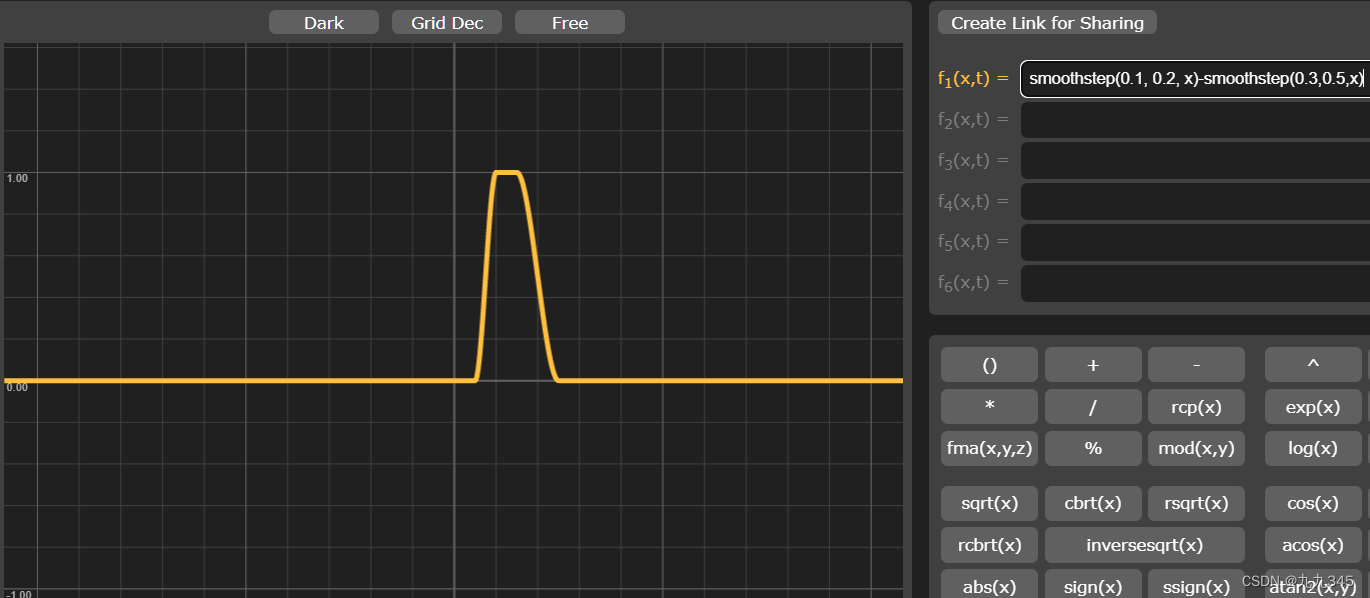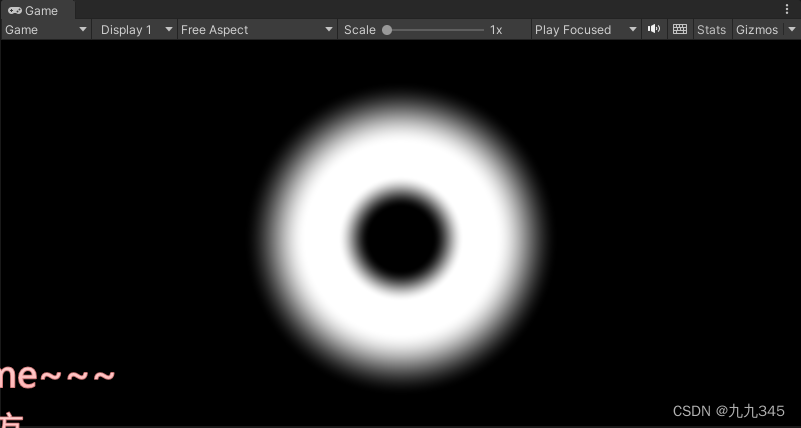# 1 step

``````// x <a,返回0
// x>=0,返回1
step(a,x);``````

``````            fixed4 frag (v2f i) : SV_Target
{
// step
fixed4 col = step(_Amount, length(i.uv - 0.5)) * _Color;
return col;
}
ENDCG``````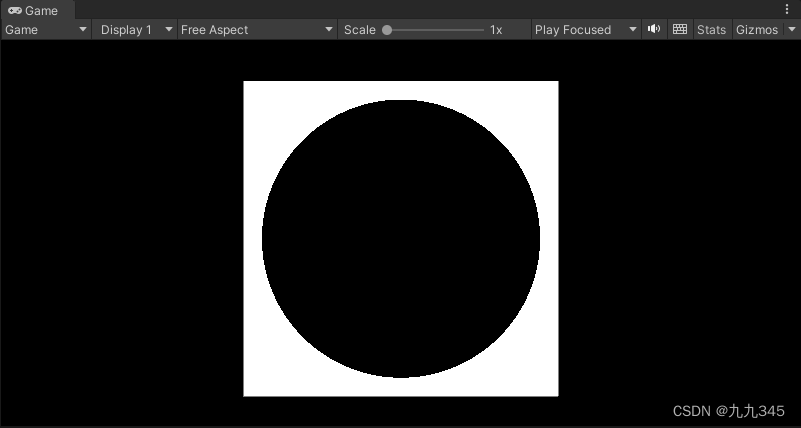# 2 lerp

``````// w是一个百分比，表示从a到b之间按照w来取值
// w = 0,返回a
// w = 1,返回b
lerp(a, b, w);``````

``````                // lerp
fixed4 col = lerp(0, 1, length(i.uv - 0.5)) * _Color;
return col;``````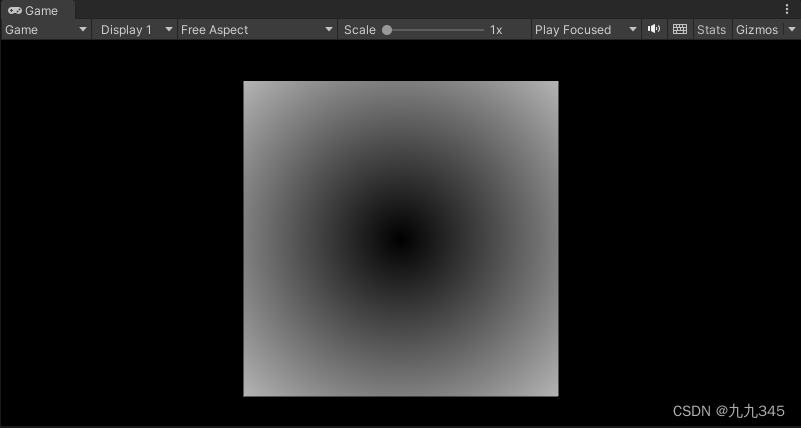# 3 smoothstep

``smoothstep(a, b, x);``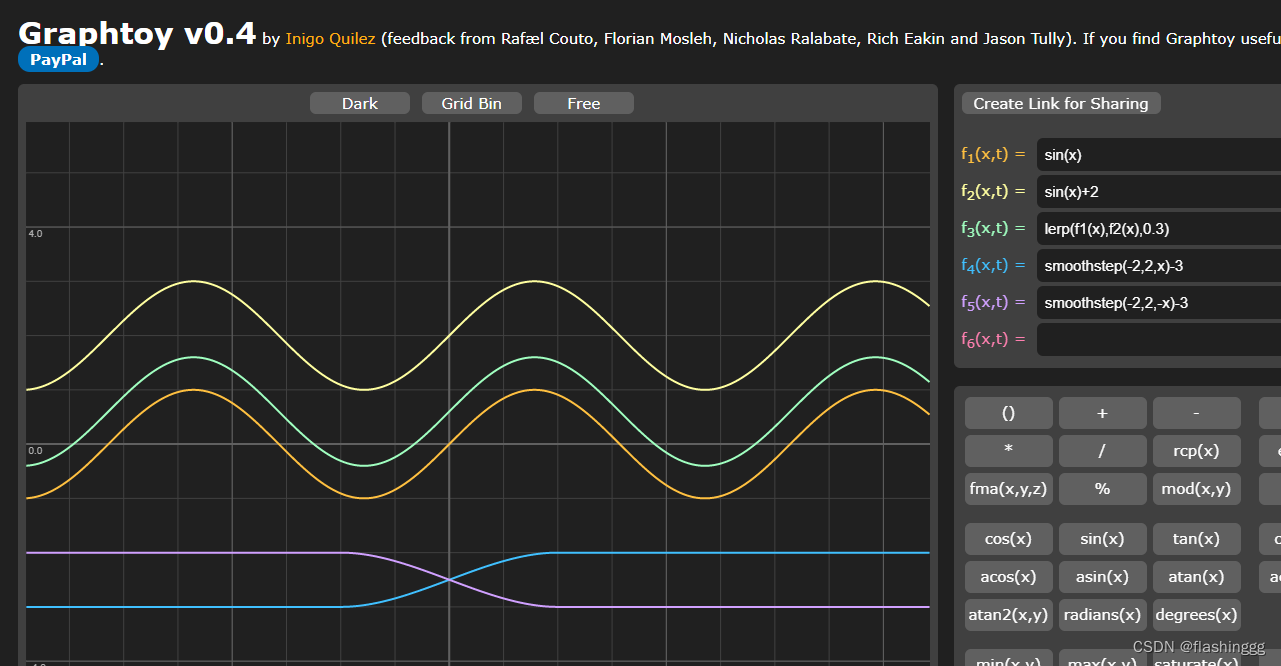``````            fixed4 singleSmoothStep(float _Start, float _End, v2f i) {
return smoothstep(_Start, _End, length(i.uv - 0.5));
}

fixed4 doubleSmoothStep(float _Start, float _End, float _Inner, float _Outer, v2f i) {
float res = smoothstep(_Start, _Inner, length(i.uv - 0.5));
float res2 = smoothstep(_Outer, _End, length(i.uv - 0.5));
return res - res2;
}

fixed4 frag (v2f i) : SV_Target
{
// step
//fixed4 col = step(_Amount, length(i.uv - 0.5)) * _Color;

// lerp
//fixed4 col = lerp(0, 1, length(i.uv - 0.5)) * _Color;

// smoothstep

//fixed4 col = singleSmoothStep(_End, _Start, i) * _Color;
fixed4 col = doubleSmoothStep(_Start, _End, _Inner, _Outer, i) * _Color;
return col;
}``````

singleSmoothStep(0.1,0.35, i)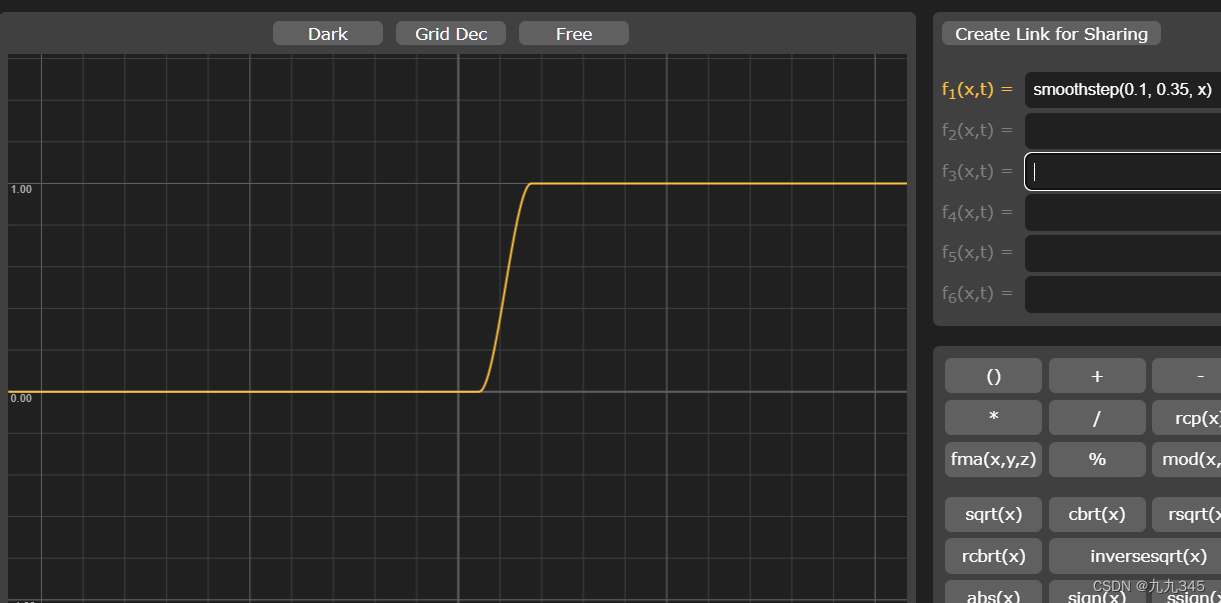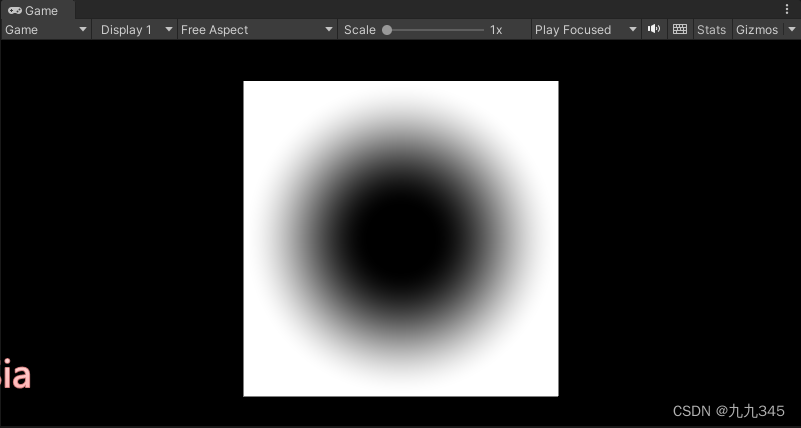singleSmoothStep(0.35,0.1, i)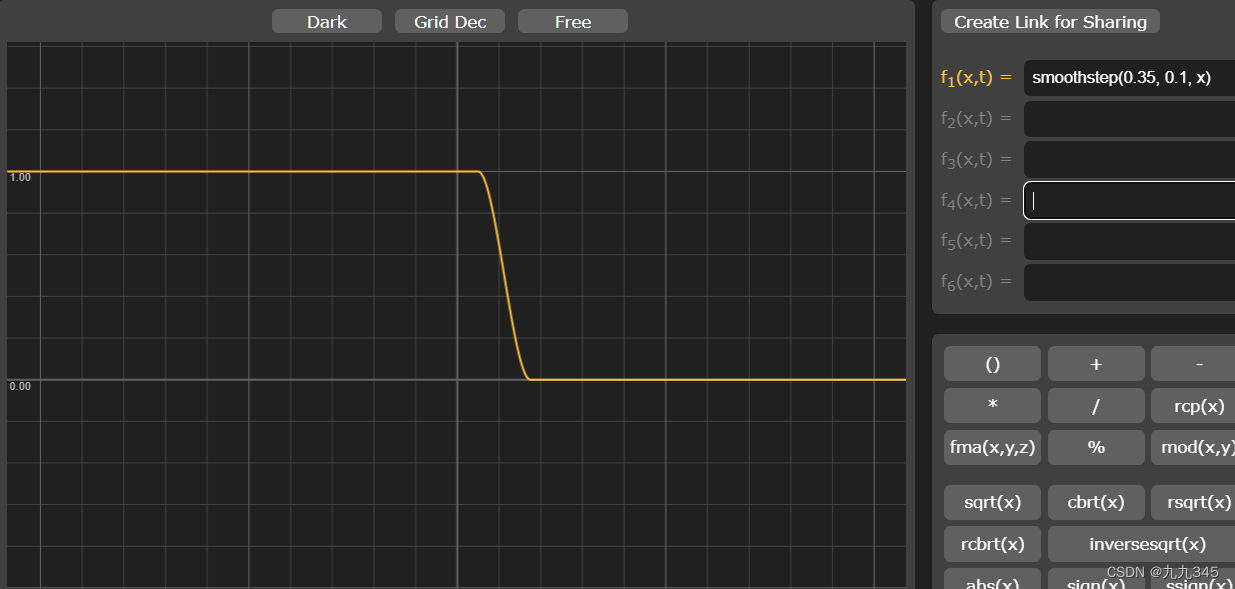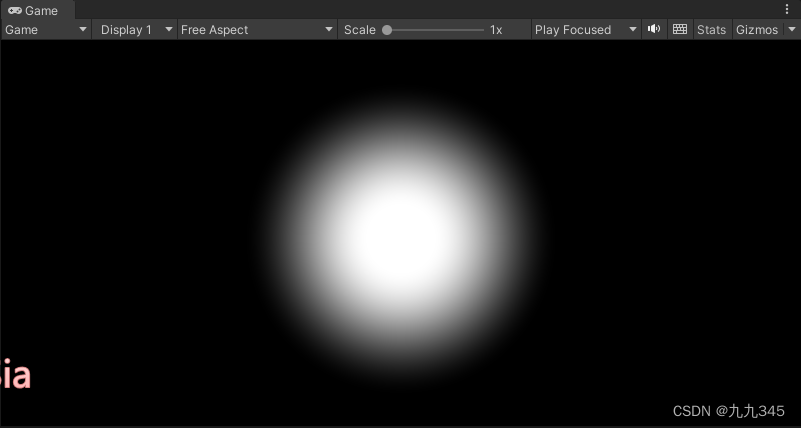doubleSmoothStep(0.1, 0.5, 0.2, 0.3, i)